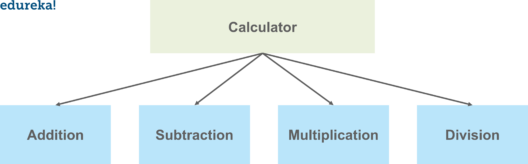# Python Modules- All You Need To know

Last updated on Nov 27,2019 2.8K ViewsPython programming language is one of the most popular language nowadays. It has numerous applications and developers are switching over to python for the implementation it provides us with. The modular programming approach where the code is broken down into separate parts is where python modules comes into picture. This article will help you understand the above topic in detail.

Following are the topics that will be covered in this blog:

## What Are Python Modules?

Modules are simply a ‘program logic’ or a ‘python script’ that can be used for variety of applications or functions. We can declare functions, classes etc in a module.

The focus is to break down the code into different modules so that there will be no or minimum dependencies on one another. Using modules in a code helps to write lesser line of codes, a single procedure developed for reuse of the code as well. It also eliminates the need to write the same logic again and again.

One more advantage of using modules is that the  programs can be designed easily, since a whole team works only on a part or module of the entire code.

Lets try to understand this with an example:

Suppose you want to make a program for a calculator. There will be operations like addition, subtraction, multiplication, division etc.

We will break the code into separate parts, we can simply create one module for all these operations or separate modules for each of the operations. And then we can call these modules in our main program logic.

The idea is to minimize the code, and if we create modules, it doesn’t mean we can only use it for this program, we can even call these modules for other programs as well.Now that we have understood the concept of modules, lets try to understand how we can create a module in python.

## How To Create Modules In Python?

Creating a module in python is similar to writing a simple python script using the .py extension. For the above example lets try to make a module for the various operations.

```def add(x,y):
return x + y

def sub(x, y):
return x - y

def prod(x, y):
return x * y

def div(x, y):
return x / y
```

Save the above code in a file Calc.py. This is how we create a module in python. We have created different functions in this module. We can use these modules in our main file, lets take a look at how we are going to use them in a program.

## How To Use Python Modules?

We will use the import keyword to incorporate the module into our program, from keyword is used to get only a few or specific methods or functions from a module. Lets see what are different methods to use a module in your program.

Lets say we have our file with a name main.py.

```
import calc as a
a = 10
b = 20

addition = a.add(a,b)
print(addition)

```

In the above code, we have created an alias using the as keyword. The output of the above code will be the addition of the two numbers a and b using the logic specified in the add function in the calc.py module.

Lets take a look at another approach.

```
from calc import *
a = 20
b = 30

print(add(a,b))

```

In the above code, we have imported all the functions using the asterisk and we can simply mention the function name to get the results.

#### Python Module Path

When we import a module, the interpreter looks for the module in the build-in modules directories in sys.path and if not found, it will look for the module in the following order:

1. Current directory
2. PYTHONPATH
3. Default directory
```
import sys

print(sys.path)
```

When you run the above code, you will get the list of directories. You can make changes in the list to create your own path.

## Built-in Modules In Python

Built-in modules are written in C and integrated with python interpreter. Each built-in module contains resources for certain specific functionalities like Operating system management, disk input/output etc.

The standard library also has many python scripts containing useful utilities.  There are several built-in modules in python at our disposal that we can use whenever we want.

To get the list of all the modules in python, you can write the following command in the python console.

```
help('modules')

```

You will get a list of all the modules in python. Below are a few modules in python.dir( ) Built-in Function

It returns a sorted list of strings containing the names defined in a module. The list contains the names of all the variables, functions, classes etc.

```
import calc

print(dir(calc))

```

You will get the list output like this:Similarly, you can get the names defined in any module using the dir( ) function.

In this blog, we have learnt about modules in python, how we can create a module and use it in the program. We have also learnt about the built in modules in python. Python programming language has enormous applications and with the use of modules, the task becomes easier, maintainable and efficient. If you wish to master your skills in python programming language you can enroll for the python certification course to kick-start your learning and become a python developer.

If you have any questions? mention them in the comments, we will get back to you.

Comments
0 CommentsREGISTER FOR FREE WEBINARThank you for registering Join Edureka Meetup community for 100+ Free Webinars each month